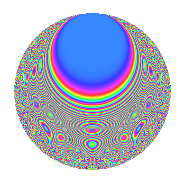# Properties

 Label 1575.2.jLevel 1575 Weight 2 Character orbit j Rep. character $$\chi_{1575}(226,\cdot)$$ Character field $$\Q(\zeta_{3})$$ Dimension 120 Sturm bound 480

# Related objects

## Defining parameters

 Level: $$N$$ = $$1575 = 3^{2} \cdot 5^{2} \cdot 7$$ Weight: $$k$$ = $$2$$ Character orbit: $$[\chi]$$ = 1575.j (of order $$3$$ and degree $$2$$) Character conductor: $$\operatorname{cond}(\chi)$$ = $$7$$ Character field: $$\Q(\zeta_{3})$$ Sturm bound: $$480$$

## Dimensions

The following table gives the dimensions of various subspaces of $$M_{2}(1575, [\chi])$$.

Total New Old
Modular forms 528 132 396
Cusp forms 432 120 312
Eisenstein series 96 12 84

## Trace form

 $$120q - 54q^{4} - 12q^{8} + O(q^{10})$$ $$120q - 54q^{4} - 12q^{8} + 10q^{11} - 4q^{13} - 12q^{14} - 42q^{16} - 12q^{17} + 6q^{19} - 16q^{22} + 10q^{23} - 2q^{28} - 20q^{31} + 22q^{32} + 48q^{34} + 14q^{37} + 22q^{38} - 16q^{41} + 16q^{43} + 64q^{44} - 6q^{47} + 2q^{49} + 44q^{52} - 20q^{53} + 6q^{56} - 2q^{58} + 30q^{59} - 6q^{61} + 36q^{62} - 16q^{67} - 8q^{68} - 8q^{71} + 22q^{73} - 24q^{74} - 76q^{76} + 64q^{77} - 22q^{82} + 24q^{83} + 6q^{86} + 76q^{88} - 16q^{89} + 32q^{91} - 76q^{92} - 54q^{94} + 8q^{97} - 10q^{98} + O(q^{100})$$

## Decomposition of $$S_{2}^{\mathrm{new}}(1575, [\chi])$$ into newform subspaces

The newforms in this space have not yet been added to the LMFDB.

## Decomposition of $$S_{2}^{\mathrm{old}}(1575, [\chi])$$ into lower level spaces

$$S_{2}^{\mathrm{old}}(1575, [\chi]) \cong$$ $$S_{2}^{\mathrm{new}}(21, [\chi])$$$$^{\oplus 6}$$$$\oplus$$$$S_{2}^{\mathrm{new}}(35, [\chi])$$$$^{\oplus 6}$$$$\oplus$$$$S_{2}^{\mathrm{new}}(63, [\chi])$$$$^{\oplus 3}$$$$\oplus$$$$S_{2}^{\mathrm{new}}(105, [\chi])$$$$^{\oplus 4}$$$$\oplus$$$$S_{2}^{\mathrm{new}}(175, [\chi])$$$$^{\oplus 3}$$$$\oplus$$$$S_{2}^{\mathrm{new}}(315, [\chi])$$$$^{\oplus 2}$$$$\oplus$$$$S_{2}^{\mathrm{new}}(525, [\chi])$$$$^{\oplus 2}$$

## Hecke Characteristic Polynomials

There are no characteristic polynomials of Hecke operators in the database